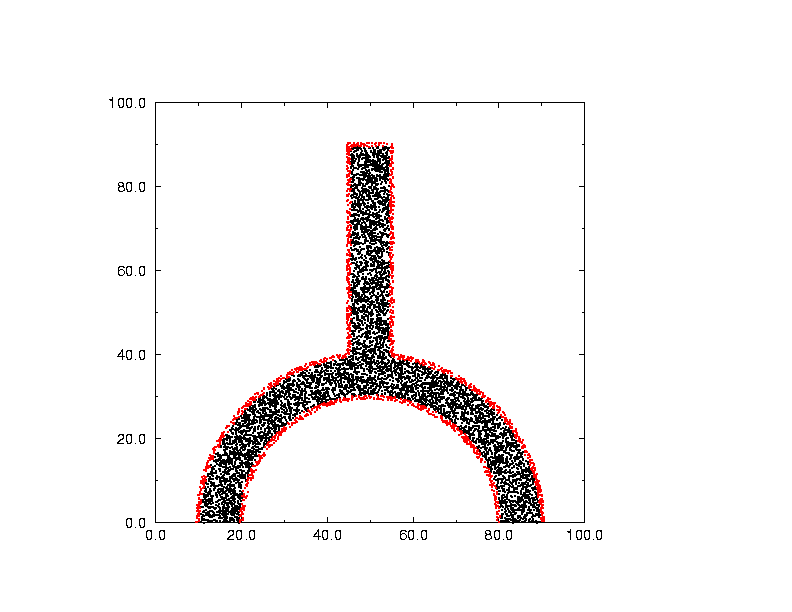# SANDIA_CVT Centroidal Voronoi Tessellations: The Release Version

SANDIA_CVT is a FORTRAN90 program which handles point placement, and moment determination, within arbitrary 2D or 3D regions.

The program was written under contract to Sandia National Laboratory,

The region is specified at a run time by an input file. The input file is processed by a large geometry package known as DIATOM. This package is apparently a variant of a package known as CTH, both of which are used at Sandia. DIATOM is written in C and FORTRAN.

Sandia expected the CVT routines to be eventually included in the body of DIATOM, so that they would simply provide an option to determine point placement and moments, as part of a larger computation.

For our testing, we included an interface with DIATOM, but we also made it possible to define the geometry of the region using a simple FORTRAN subroutine that simply says "yes, this point is in the region". By cutting out a few calls, the interface to DIATOM can be severed, and a standalone package can be produced for other purposes.Here is a picture of the test region. The red points lie on the boundary and the black points lie within. These data points were not generated by the Voronoi code. Instead, 50,000 uniformly distributed points were generated in the bounding box, and then those points in or on the region were selected. It's hard to tell from this picture, but a set of points selected from a uniform distribution tend to have voids and clusters, which will NOT occur in a Voronoi method.

### Languages:

SANDIA_CVT is available in a FORTRAN90 version.

### Related Data and Programs:

CVT, a FORTRAN90 library which computes CVT's.

CVT, a dataset directory which contains a variety of CVT datasets.

CVT_DATASET, a FORTRAN90 program which computes CVT's.

LCVT, a FORTRAN90 library which computes a latinized Centroidal Voronoi Tessellation.

### Reference:

1. Franz Aurenhammer,
Voronoi diagrams - a study of a fundamental geometric data structure,
ACM Computing Surveys,
Volume 23, Number 3, pages 345-405, September 1991.
2. John Burkardt, Max Gunzburger, Janet Peterson and Rebecca Brannon,
User Manual and Supporting Information for Library of Codes for Centroidal Voronoi Placement and Associated Zeroth, First, and Second Moment Determination,
Sandia National Laboratories Technical Report SAND2002-0099,
February 2002.
3. Qiang Du, Vance Faber, and Max Gunzburger,
Centroidal Voronoi Tessellations: Applications and Algorithms,
SIAM Review, Volume 41, 1999, pages 637-676.
4. John Halton,
On the efficiency of certain quasi-random sequences of points in evaluating multi-dimensional integrals,
Numerische Mathematik,
Volume 2, 1960, pages 84-90.
5. Lili Ju, Qiang Du, and Max Gunzburger,
Probabilistic methods for centroidal Voronoi tessellations and their parallel implementations,
Parallel Computing,
Volume 28, 2002, pages 1477-1500.

### Source Code:

SANDIA_CVT is the source code for the CVT library.

• cvt.f90, the program library;
• diatom_setup.c, some C interface routines that set up an interface with the DIATOM library;

DIATOM_SETUP is the source code for routines, written in C, that set up an interface with the DIATOM library.

CVT_MAIN is the text of a sample main program that calls the SANDIA_CVT library. It does not require the DIATOM library.

### List of Routines:

• BIN_PREPROCESS organizes the preprocessing step for bins.
• BIN_TO_R8_EVEN2 returns the limits for a given "bin" in [A,B].
• BIN_TO_R8VEC_EVEN3 returns the limits for a given R8VEC "bin" in [A,B].
• CVT_ITERATION takes one step of the CVT iteration.
• FIND_CLOSEST finds the Voronoi cell generator closest to a point X.
• GENERATOR_INIT initializes the Voronoi cell generators.
• I4_TO_HALTON_VECTOR computes an element of a vector Halton sequence.
• INDEX_BOX2_NEXT_2D produces indices on the surface of a box in 2D.
• INDEX_BOX2_NEXT_3D produces indices on the surface of a box in 3D.
• POINTS_NEAREST_POINT_BINS3_2D finds the nearest point to a given point in 2D.
• POINTS_NEAREST_POINT_BINS3_3D finds the nearest point to a given point in 3D.
• QUALITY computes some quality measures for a set of points in a region.
• R8_TO_BIN_EVEN2 determines the appropriate "bin" for C in [A,B].
• R82VEC_BIN_EVEN3 bins a D2 array into evenly spaced bins.
• R82VEC_BINNED_REORDER2 reorders a binned D2 data vector.
• R82VEC_BINNED_SORT_A2 sorts each bin of a D2 binned data vector.
• R83VEC_BIN_EVEN3 bins a D3 array into evenly spaced bins.
• R83VEC_BINNED_REORDER2 reorders a binned D3 data vector.
• R83VEC_BINNED_SORT_A2 sorts each bin of a D3 binned data vector.
• R8COL_PART_QUICK_A reorders the columns of an array as part of a quick sort.
• R8COL_SORT_QUICK_A ascending sorts the columns of a table using quick sort.
• R8MAT_DET_2D computes the determinant of a 2 by 2 matrix.
• R8MAT_DET_3D computes the determinant of a 3 by 3 matrix.
• R8VEC_EQ is true if every pair of entries in two vectors is equal.
• R8VEC_GT == ( A1 > A2 ) for double precision vectors.
• R8VEC_LT == ( A1 < A2 ) for double precision vectors.
• R8VEC_SWAP swaps the entries of two double precision vectors.
• R8VEC_TO_BIN_EVEN3 determines the appropriate "bin" for a R8VEC value.
• RANDOM_INITIALIZE initializes the FORTRAN 90 random number seed.
• REGION_SAMPLER returns a sample point in the physical region.
• TIMESTAMP prints the current YMDHMS date as a time stamp.
• VCM calculates Voronoi cell volumes, centroids and second moments.

You can go up one level to the FORTRAN90 source codes.

Last revised on 31 January 2010.